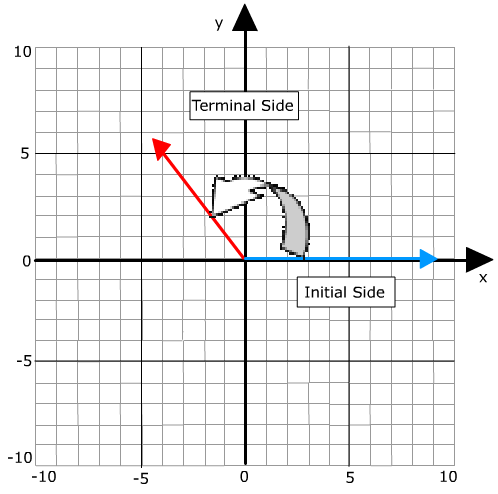# Standard Position of an Angle - Initial Side - Terminal Side

An angle is in standard position in the coordinate plane if its vertex is located at the origin and one ray is on the positive x-axis. The ray on the x-axis is called the initial side and the other ray is called the terminal side.The initial side is where the angle starts and the terminal side is the ray where the measurement of the angle stops, therefore the terminal side defines the angle and if the vertex is at the origin (0, 0) then the angle will be in Standard Position.

 Related Links: Math Trigonometry Introduction to the Six Trigonometric Functions (Ratios)

To link to this Standard Position of an Angle - Initial Side - Terminal Side page, copy the following code to your site: